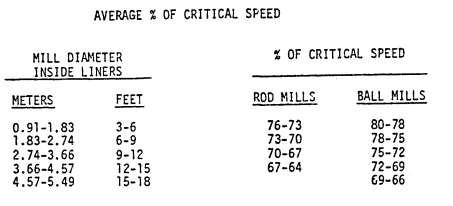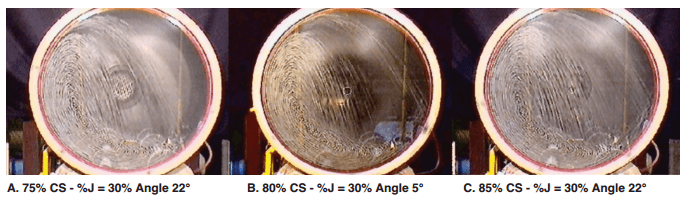# Ball Mill Critical Speed

A Ball Mill Critical Speed (actually ball, rod, AG or SAG) is the speed at which the centrifugal forces equal gravitational forces at the mill shell’s inside surface and no balls will fall from its position onto the shell.The imagery below helps explain what goes on inside a mill as speed varies.

Use our online formulaThe mill speed is typically defined as the percent of the Theoretical Critical Speed, often abbreviated as %Cs.
The Theoretical Critical Speed (Cs) of rotation is the speed (in RPM) at which an infinitely small particle will cling to the inside of the liners for a complete revolution. The percent of critical speed is the ratio (expressed as a percentage) of the actual mill speed and the Theoretical Critical Speed of that mill.  The critical speed of a rotating mill is the RPM at which a grinding medium will begin to “centrifuge”, namely will start rotating with the mill and therefore cease to carry out useful work.

Ball mills have been successfully run at speeds between 60 and 90 percent of critical speed, but most mills operate at speeds between 65 and 79 percent of critical speed.

Rod mills speed should be limited to a maximum of 70% of critical speed and preferably should be in the 60 to 68 percent critical speed range.

Pebble mills are usually run at speeds between 75 and 85 percent of critical speed.

The black dot in the imagery above represents the centre of gravity of the charge.

http://www.pauloabbe.com/size-reduction/resources/mill-speed-critical-speed

The Formula derivation ends up as follow: Critical Speed is: Nc =76.6(D0.5)

where:

• Nc is the critical speed,in revolutions per minute,
• D is the mill effective inside diameter, in feet.

Example: a mill measuring 11’0” diameter inside of new shell liners operates at 17.3 rpm. Critical speed is

C.S. = 76.63 / 11^0.5 = 23.1 rpm

Ball and SAG Mills are driven in practice at a speed corresponding to 60-81% of the critical speed, the choice of speed being influenced by economical considerations. Within that range the power is nearly proportional to the speed.

Mill rotating speed impacts grinding rates. A great little paper about this is ” Effect of Fraction of Mill Critical Speed on Kinetic Breakage “.

This video should the working principle of a ball mill and the ball action at various speed near up/down from critical.

You’ll find a good on-line tool for ball mill critical speed calculation based on the above formula.